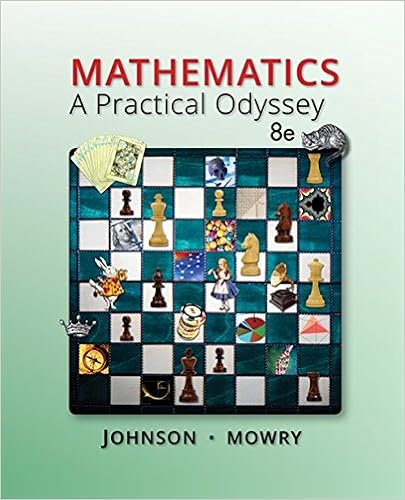# Factorial MANOVA Q & A.doc - Factorial(2 x 3 MANOVA Using...

• Notes
• 2
• 100% (4) 4 out of 4 people found this document helpful

This preview shows page 1 - 2 out of 2 pages.

##### We have textbook solutions for you!
The document you are viewing contains questions related to this textbook.The document you are viewing contains questions related to this textbook.
Chapter 3 / Exercise 34
Mathematics: A Practical Odyssey
Johnson/MowryExpert Verified
Factorial (2 x 3) MANOVA Using SPSS1.Is there a sufficient correlation between the dependent variables to justify the use of MANOVA?2.Was the assumption of Equality of Covariance Matrices violated? Explain.3.Is there a statistically significant multivariate interaction effect?Identify the dependent variable(s) of this interaction effect. 4.What would be the proper follow-up tests for a statistically significant interaction effect?5.Identify the proper post hoc analyses for any statistically significant univariate effects. Explain your answer.6.Is there a statistically significant multivariate gender effect on the dependent variate?7.Why would a researcher conduct a MANOVA instead of several ANOVAs? 8.Write a Results section for this research.1.Is there a sufficient correlation between the dependent variables to justify the use of MANOVA?YES! THE DEPENDENT VARIABLES ARE BOTH CONCEPTUALLY AND STATISTICALLY (r = .513) RELATED.
##### We have textbook solutions for you!
The document you are viewing contains questions related to this textbook.The document you are viewing contains questions related to this textbook.
Chapter 3 / Exercise 34
Mathematics: A Practical Odyssey
Johnson/MowryExpert Verified
•••